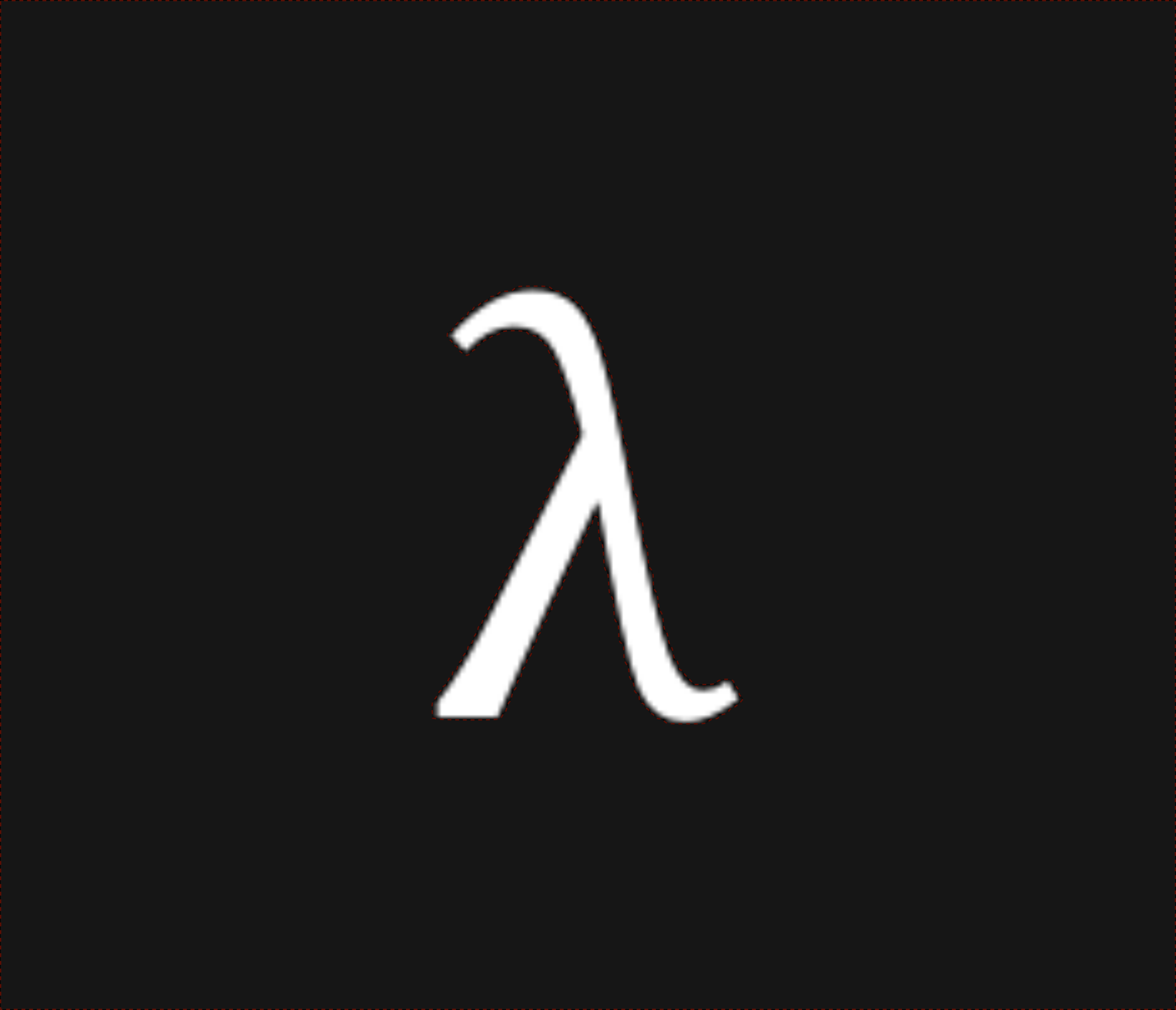nixpulvis

## Set Builder Notation 502 words published on March 16, 2020.

I’m not a fan of set builder notation, and let me explain why…

I mean, it beats a lot of other things mathematicians were probably doing before they agreed to it, but that could be said about pretty much anything. Just look at how many people love writing Python, and how god awful that language is. I’d expect a bit more from math notation, but I digress.

We can read a set $$\{\ e : x \in \mathbb{D}\ \}$$ as “$$\ e$$ such that $$x$$ is in $$\mathbb{D}\,$$”. Here $$e$$ is an expression which has $$x$$ bound within it.

To fully explain my position, let’s start by looking at a good use of set builder notation.

$A = \{\ 2x^2 : x \in \mathbb{R} \ \} \\$

Here we lead with the interesting stuff, being the $$2x^2$$, and brush the fact that $$x \in \mathbb{R}$$ under the rug. This is more or less OK, because you don’t need to be thinking about what kind of number $$x$$ is to understand the equation in this case. It’s purely a formality, which may be necessary to a larger context.

However, things start getting interesting quickly.

$\{\ \sqrt{x} - 2 : x ∈ [1,10) \ \}$

Suddenly the domain of the set and $$x$$ aren’t necessarily the same, yet we would probably assume something reasonable, right? These must all be $$\in \mathbb{R}$$, or something. Still we’ve tried to lead with what we care about, the $$\sqrt{x} - 2$$ in this case. But suddenly the right side matters a lot more.

Next, we start calling functions on the right side.

$\{\ \sqrt{x} : x \in filter([0, ∞), odd?) \ \}$

I like to think someone would stop at this point and restructure the equations. Why am I operating on $$x$$ in $$\sqrt{x}$$ before I even know what $$x$$ is?! A quick glance, and you don’t even notice it anymore, it’s lost in the noise on the right.

Finally, we come to what Python starts letting you do with their surprisingly beloved array comprehensions, which are basically just set builder notation in ASCII. In math, at least we can argue about the evaluation order a little bit, but in Python this is just downright wrong!

[bar(x) for x in foo(x)]


The function foo will execute before the function bar, and this is not entirely clear by reading the source. In fact, this makes me wonder what other things in Python evaluate backwards…

One more thing, what about chained array comprehensions?

[int(b) for x in range(0, 10) for b in bin(x)[2:]]


My head hurts looking at this code. Where is x bound? Where is b bound? It’s all so unclear, it really makes me wonder…